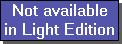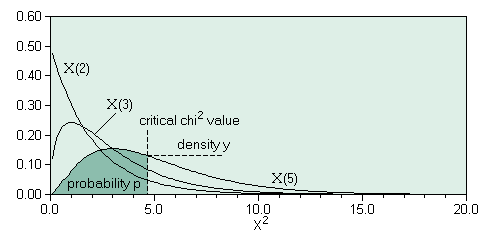The SDL Component Suite is an industry leading collection of components supporting scientific and engineering computing. Please visit the SDL Web site for more information....## Chi2DistriIntegralUnit: SDL_statis Class: none Declaration: function Chi2DistriIntegral (chi2: double; df: integer): double;

The function Chi2DistriIntegral returns the probability p for a given critical value chi2 , and a given number of degrees of freedom df . The probability p is equal to the integral of the chi-square-distribution between zero and the value of the parameter Chi2 . The returned value may vary between 0.0 and 1.0.Hint 1: The calculated value is exact to about 8 decimal places.

 Hint 2: The function Chi2DistriIntegral calculates the integral from 0.0 to the Chi-Square value. This differs from the convention of how the integral of the Chi-Square distribution is used in many statistical packages, which tabulate the integral between +infinite and the Chi-Square value. Thus you have to use 1-Chi2DistriIntegral to get comparable results. For example, in order to calculate the function CHIDIST(x,df) of Microsofts Excel® you have to use 1-Chi2DistriIntegral(x,df).

Last Update: 2020-Aug-04